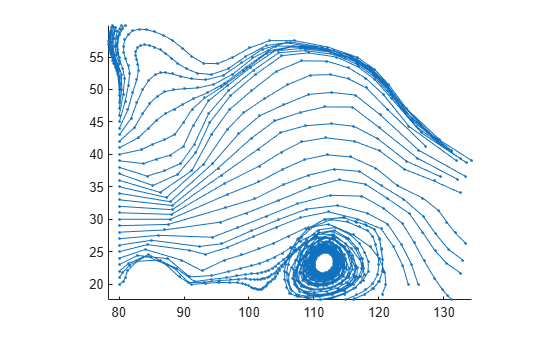# interpstreamspeed

Interpolate stream-line vertices from flow speed

## Syntax

```interpstreamspeed(X,Y,Z,U,V,W,vertices) interpstreamspeed(U,V,W,vertices) interpstreamspeed(X,Y,Z,speed,vertices) interpstreamspeed(speed,vertices) interpstreamspeed(X,Y,U,V,vertices) interpstreamspeed(U,V,vertices) interpstreamspeed(X,Y,speed,vertices) interpstreamspeed(speed,vertices) interpstreamspeed(...,sf) vertsout = interpstreamspeed(...) ```

## Description

`interpstreamspeed(X,Y,Z,U,V,W,vertices)` interpolates streamline vertices based on the magnitude of the vector data `U`, `V`, `W`.

The arrays `X`, `Y`, and `Z`, which define the coordinates for `U`, `V`, and `W`, must be monotonic, but do not need to be uniformly spaced. `X`, `Y`, and `Z` must have the same number of elements, as if produced by `meshgrid`.

`interpstreamspeed(U,V,W,vertices)` assumes `X`, `Y`, and `Z` are determined by the expression

`[X Y Z] = meshgrid(1:n,1:m,1:p) `

where `[m n p] = size(U)`.

`interpstreamspeed(X,Y,Z,speed,vertices)` uses the 3-D array `speed` for the speed of the vector field.

`interpstreamspeed(speed,vertices)` assumes `X`, `Y`, and `Z` are determined by the expression

`[X Y Z] = meshgrid(1:n,1:m,1:p) `

where `[m n p]=size(speed)`.

`interpstreamspeed(X,Y,U,V,vertices)` interpolates streamline vertices based on the magnitude of the vector data `U`, `V`.

The arrays `X` and `Y`, which define the coordinates for `U` and `V`, must be monotonic, but do not need to be uniformly spaced. `X` and `Y` must have the same number of elements, as if produced by `meshgrid`.

`interpstreamspeed(U,V,vertices)` assumes `X` and `Y` are determined by the expression

`[X Y] = meshgrid(1:n,1:m) `

where `[M N]=size(U)`.

`interpstreamspeed(X,Y,speed,vertices)` uses the 2-D array `speed` for the speed of the vector field.

`interpstreamspeed(speed,vertices)` assumes `X` and `Y` are determined by the expression

`[X Y] = meshgrid(1:n,1:m)`

where `[M,N]= size(speed)`.

`interpstreamspeed(...,sf)` uses `sf` to scale the magnitude of the vector data and therefore controls the number of interpolated vertices. For example, if `sf` is 3, then `interpstreamspeed` creates only one-third of the vertices.

`vertsout = interpstreamspeed(...)` returns a cell array of vertex arrays.

## Examples

collapse all

Draw streamlines using the vertices returned by `interpstreamspeed`. Dot markers indicate the location of each vertex. Streamlines with widely spaced vertices indicate faster flow. Those with closely spaced vertices indicate slower flow.

```load wind [sx,sy,sz] = meshgrid(80,20:1:55,5); verts = stream3(x,y,z,u,v,w,sx,sy,sz); iverts = interpstreamspeed(x,y,z,u,v,w,verts,0.2); sl = streamline(iverts); set(sl,'Marker','.'); axis tight; view(2); daspect([1 1 1]);```Plot streamlines to show vertex spacing as it changes with the gradient along the streamline.

```z = membrane(6,30); [u,v] = gradient(z); pcolor(z); hold on [verts,averts] = streamslice(u,v); iverts = interpstreamspeed(u,v,verts,15); sl = streamline(iverts); set(sl,'Marker','.'); shading interp axis tight view(2); daspect([1,1,1]); hold off```